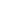# Mulch Calculator

Mulch Calculator Form

This calcuator determines mulch needs based on known dbh measurements of a given tree. The presumed area to mulch is based on the city of Austin's definition of 1/2 critical root zone. This measurement is calculated by converting trunk diameter measurement to feet and dividing by 2. For example, a 20" diameter tree would have a mulch zone that extends 10' radius out from the center of the trunk. If you have multiple trees to mulch, this tool will aggregate the calculations to give you the total yards of mulch needed. If you want to draw a custom mulch zone on a map for less structured calculations, try using our other mulch calculator.